Courses

# Test: Soil Mechanics- 3

## 10 Questions MCQ Test RRB JE for Civil Engineering | Test: Soil Mechanics- 3

Description
This mock test of Test: Soil Mechanics- 3 for Civil Engineering (CE) helps you for every Civil Engineering (CE) entrance exam. This contains 10 Multiple Choice Questions for Civil Engineering (CE) Test: Soil Mechanics- 3 (mcq) to study with solutions a complete question bank. The solved questions answers in this Test: Soil Mechanics- 3 quiz give you a good mix of easy questions and tough questions. Civil Engineering (CE) students definitely take this Test: Soil Mechanics- 3 exercise for a better result in the exam. You can find other Test: Soil Mechanics- 3 extra questions, long questions & short questions for Civil Engineering (CE) on EduRev as well by searching above.
QUESTION: 1

### Except which among the following properties, the permeability of soil increases?

Solution:

Permeability (k) =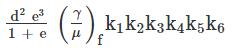· More will be the representative grain size, more will be the permeability of the soil.

· Higher is the degree of saturation Higher is the permeability of the medium as lesser is the resistance available to the flow of fluid in the form of air blocks

· More will be the unit weight of fluid, more will be permeability of the medium

· Higher is the presence of entrapped gases, lower is the permeability of the medium as higher is the resistance available to the flow of the fluid.

QUESTION: 2

### Which one of the following is correct in respect of pore water pressure u and effective stress σ, in the soil just below the bottom of a pond due to a 2 m rise in water level in the pond?

Solution:

The rise in water level in the pond causes equal increase in total stress and pore pressure so effective stress does not change. The pore pressure will increase by 20 kN/m2.

QUESTION: 3

### A fully saturate capillary zone of thickness 2 m exists above the water table in a fine silty sand deposit. Water is the pore water pressure at 1.5 m above the water table?

Solution:

Above the water table pore pressure will be negative

Pore pressure at 1.5 m above the WT

= - γwz = - 10 × 1.5 = -15 kN/m2.

QUESTION: 4

According to Darcy’s law for flow through porous media, the velocity is proportional to

Solution:

Darcy established that the flow occurring per unit time is directly proportional to the head causing flow and the area of cross – section of the soil sample but is inversely proportional to the length of the soil sample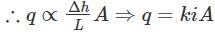Dividing both sides by A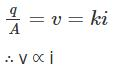QUESTION: 5

In the figure shown above, which one of the following correctly represents effective stress at C? (Symbols have the usual meanings)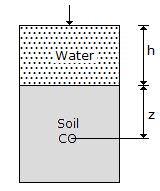Solution:

Total stress at C = γwh + γsatZ

Pore pressure at C = γw(h + z)

Effective stress = (γsat - γw)Z = γsubZ

*Answer can only contain numeric values
QUESTION: 6

Previous sand is used in the foundation of a masonry dam such that upward hydraulic gradient permissible on it will be 0.345. Determine the factor of safely required against quicksand condition, if porosity and a specific gravity of soil solids are 0.40 and 2.65 respectively.

Solution:

Concept:

Critical hydraulic Gradient, icr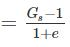Factor of safety (F.O.S) =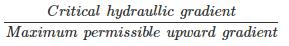Calculation: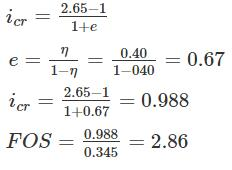*Answer can only contain numeric values
QUESTION: 7

For a capillary rise in a soil mass, void size of which is assumed to be 20% of the effective size of the particle (D10), surface tension is observed to be 73 × 10-3 N/m. Calculate the value of empirical constant given by Hazen in cm2 void ratio of the soil sample is 0.30. Take γw = 10 kN/m3

Solution:

Height of Capillary rise =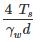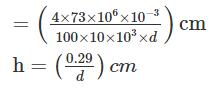Height of Capillary rise given by Hazen: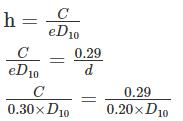C = 0.435 cm2

QUESTION: 8

Steady-state seepage is taking place through a soil element at Q, 2 m below the ground surface immediately downstream of the toe of an earthen dam as shown in the sketch. The water level in a piezometer installed at P, 500 mm above Q, is at the ground surface. The water level in a piezometer installed at R, 500 mm below Q, is 100 mm above the ground surface. The bulk saturated unit weight of the soil 18 kN/m3 and the unit weight of water is 9.81 kN/m3. The vertical effective stress (in kPa) at Q is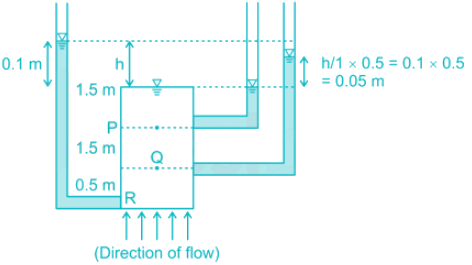Solution:

Explanation : Pore water pressure, µ = γw. h.

µP = γw × 1.5 m

µR = γw × (2.5 + 0.1) = γw × 2.6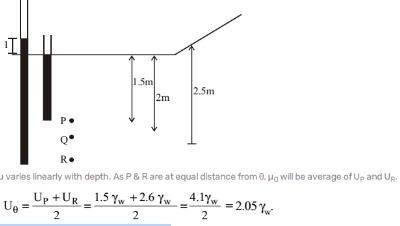Effective stress, σ = σ – U

at Q = γsat. h – UQ.

= 18 × 2 – 2.05 × 9.81 = 15. 89 kPa

*Answer can only contain numeric values
QUESTION: 9

A lake of depth 15 m is filled with water up to a depth of 10 m from the Bottom of the lake. Soft clay is present below the bottom of the lake up to a certain depth of more than 20 m. Submerged unit weight of the clay is given as 9.5 kN/m3. Take γw = 10 kN/m3. Calculate the effective Stress (kN/m2) at a depth of 15 m below the bottom of the lake.

Solution: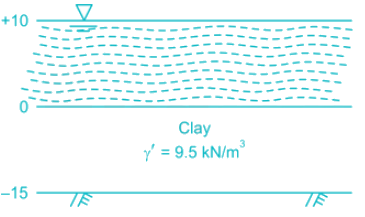γ’ = γsat - γw

γsat = γ’ + γ= 9.5 + 10 = 19.5 kN/m3

σ’ = σ - u

At at depth of 15 m below lake

σ = γ× 10 + γsat × 15

σ = 10 × 10 + 19.5 × 15

σ = 392.5 kw/m2

u = 25 × γw = 25 × 10 = 250 kN/m2

= σ - 4

= 392.5 - 250

= 142.5 kN/m2

QUESTION: 10

Assuming that a river bed level does not change and the depth of water in the river was 10m, 15m, and 8m during the months of February, July and December respectively of a particular year. The average bulk density of the soil is 20 kN/m3. The density of water is 10 kN/m3. The effective stress at a depth of 10 m below the river bed during these months would be

Solution:

Since the river bed level does not change and the depth of water is changing only above the river bed, the effective stress below the river bed will not change.

Total stress at a depth of 10 m below river bed.

σ = 20 × 10 = 200 kN/m2

Pore water pressure,

u = 10 × 10 = 100 kN/m2

∴ Effective stress,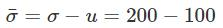= 100 kN/m2

Thus effective stress will be 100 kN/m2 each on the month of February, July and December.